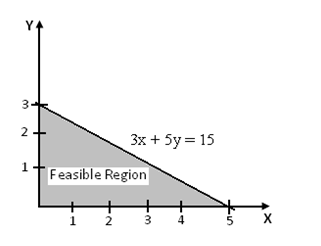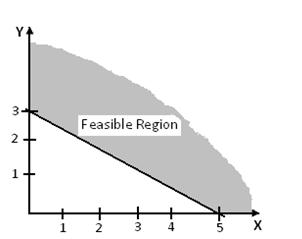#### Solve LPP by Graphical Solution Procedure, Sample Assignments

1. Work out or solve 3x + 5y < 15 graphically

Write the known constraint in the form of equation, that is 3x + 5y = 15

Put x=0, then value of y=3

Put y=0, then value of x=5

Thus, the coordinates are (0, 3) and (5, 0). Hence these points are joined to get a straight line as present in the graph.

Place x=0, y=0 in the given constraint then

0<15, the condition is true. (0, 0) is solution closer to origin. Thus shade the region below that line, which is referred to the feasible region.2. Work out or Solve 3x + 5y >15

Solution

Write the given constraint in the form of equation, that is 3x + 5y = 15

Place x=0, then y=3

Place y=0, then x=5

Now the coordinates are (0, 3) and (5, 0)

Place x =0, y =0 in the known constraint, the condition becomes false i.e. 0 > 15 is false.

Consequently the region does not have (0, 0) as solution. The feasible region stays on the exterior part of the line as presented in the graph.Need Graphical Solution Procedure Assignment Help - Homework Help?

Do you have incomplete assignments and problems in Graphical Solution Procedure ? Are you not finding proper answers for your complex Graphical Solution Procedure questions in operation research? We at www.tutorsglobe.com offer Graphical Solution Procedure assignment help, Graphical Solution Procedure homework help and answering questions and projects help in operation research. TutorsGlobe finest service provides you ability to solve all kind of future problems in Graphical Solution Procedure with no more external help. We offer step by step answers for your Graphical Solution Procedure problems and questions.

Why TutorsGlobe for Graphical Solution Procedure Assignment Help

Unique methodology and step by step answers

Conceptual and easy approach to solve Graphical Solution Procedure problems

Punctuality and Responsibility of Team and Staff

Experienced tutor's guidance

Best support materials for assignment help

100% authentic solution and guaranteed satisfaction